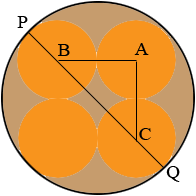SEARCH HOMEMath Central Quandaries & QueriesQuestion from KRIS, a student: YOU ARE PaCKAGING 4 EQUAL SIZED PAINT CANS WITH A RADIUS 8CM AND A HEIGHT OF 20CM, IN A CIRCULAR CONTAINER.What is the surface area of the packing container?Hi Kris,

Here is my view of the top of the packing container.A, B and C are centers of three of the paint cans. What is the length of the line segment BA? What is the length of the line segment AC? Triangle ABC is a right triangle. What is the length of the hypotenuse CA? (Ask Pythagoras.)

What is the length of he line segment PQ? this is the diameter of the cylindrical container. What is the surface area of a cylinder?

PennyMath Central is supported by the University of Regina and The Pacific Institute for the Mathematical Sciences.